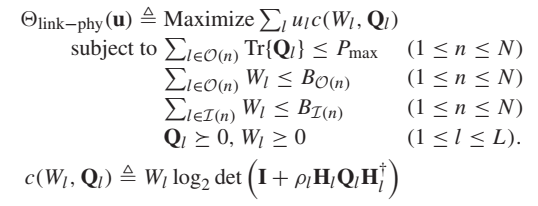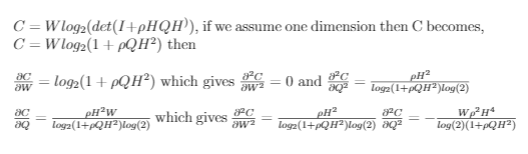# Cannot perform the operation: {real affine} .* {concave}

Hello everyone, I am pretty new to cvx. I faced the problem of solving the following optimization problem. Please help me to formulate it properly in cvx. Thanks in advance.My MATLAB code is (I just mention the necessary lines which needs to be corrected),
cvx_begin
variable WW(1,L) nonnegative
variable QQ(M,M,L) semidefinite

% eqn 3.1
for i=1:L
CC(i)=(WW(i)./log(2))*log_det(eye(M)+rho(i)*H(:,:,i)*QQ(:,:,i)*H(:,:,i)’);
end

cvx_end

You are multiplying the variable `WW(i)` with the concave expression `log_det(...)`. Is that product jointly concave in `W(I)` and `QQ(:,:,i)` given the other constraints? Can you show us a proof? But it appears to me to be neither convex nor concave even in one dimension, even with the nonegativity (semidefinite) constraints on W and Q.

According to the authors, c(W_l,Q_l) is a monotonically increasing concave function for W_l > 0 and
Q_l \succcurlyeq 0. Noted that, Q is only semidefinite positive variable here.

Show us the proof.

I forgot to post this link before.You are right about the function. So I tried primal decomposition method by fixing Q to get W and then using this W for getting Q but results are not good enough.

Can you suggest any MATLAB based solver which can take care of this problem (here H is complex matrix and Q is semidefinite positive variable)?

In FMNCON, you would need to implement the SDP constraint indriectly, for instance by a parameterization such as `X'*X` in place of the psd matrix `Q`. But in doing so, you may introduce spurious stationary points (not present in the original problem), which can cause significant difficulty. I think YALMIP can handle the complex numbers for you by converting to an equivalent real variable problem before calling FMINCON.